# How to use the IF function in ExcelThe IF function in Excel is undoubtedly one of the most useful functions there is. It is used to perform logical tests on spreadsheet data, and return different values or carry out different actions depending on whether the logical tests evaluate as TRUE or FALSE.

You can use the IF function on its own, in a group of multiple IF functions (nested IFs), or together with other logical functions like OR and AND.

The logic behind the IF function is an if-then-else structure. The IF statement first evaluates a cell (or multiple cells) to determine if it matches the criteria of the logical expression. Based on the result, the IF statement then returns a value or carries out an action (e.g. a calculation or another function). The value returned or action generated will depend on whether the input cell evaluates as TRUE or FALSE when tested with the logical expression.

In short, you tell the function that if the cell meets the criteria, do this, otherwise do that. You set the criteria and the desired results. These results can be whatever you want, which is why the IF function is so versatile.

The following flow chart illustrates the process.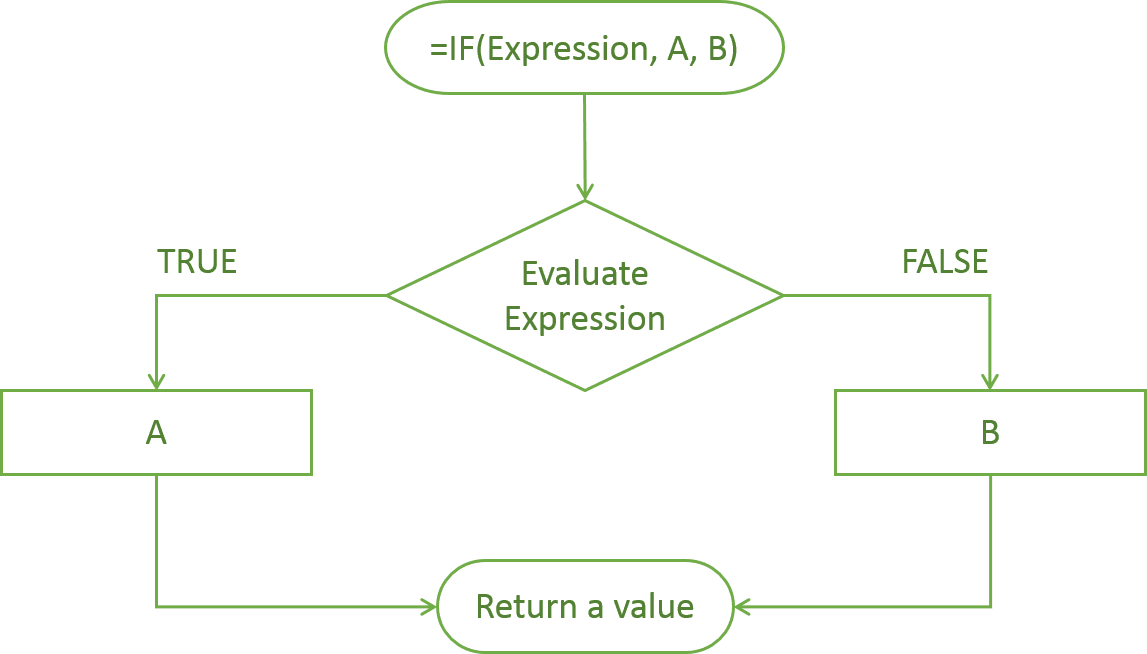### IF function Excel

#### Syntax

=IF(logical_expression, value_if_true, value_if_false)
• logical_expression – an expression or reference to a cell containing an expression that has a logical value such as TRUE or FALSE.
• value_if_true – the value that the function returns if the logical_expression is TRUE. This can be a number, text, or even another function that returns a value. You can also embed another IF statement in here: this is known as a nested IF.
• value_if_false – the value that the IF function returns if the logical_expression is FALSE. Similar to the value_if_true, this can be a number, text or another function that returns a value. It can also be a nested IF. Note that this is an optional input and if you leave it out you will get a blank value when the expression is FALSE.

#### How to use the IF function in Excel

Now let me show you the IF function in action. The first example is fairly simple: I have some data on dietary preferences of a group of people, and I’d like to use the IF function to generate certain answers about the data.

Take a look at the screenshot below. I use the IF function to determine whether or not a person’s favourite food is pizza. The formula I use to do that is =IF(C2=”Pizza”,”Yes”,”No”).

Even though this is a very simple example of the IF function, I’ll quickly break down what’s going on in the formula.

The first part within the brackets is the logical expression. This expression asks the function to evaluate whether the cell (C2 in this case) is equal to “Pizza”. Note that you have to put quotation marks around text strings.

The “Yes” and “No” are the value_if_true and value_if_false. This means that I am telling the function: If the cell says Pizza, return Yes, otherwise return No. You can see the results for yourself: the two pizza lovers got a yes, and the rest got a no.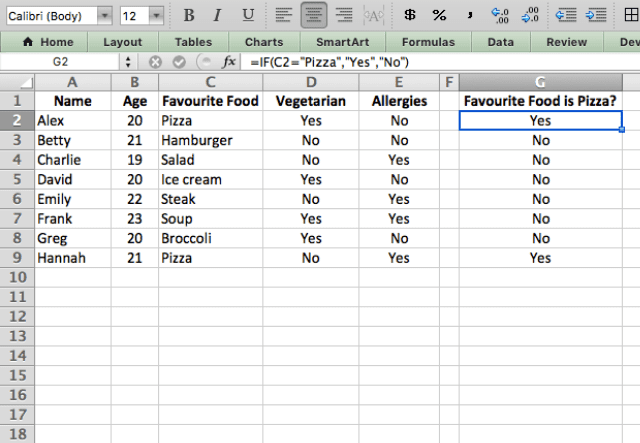Now I’ll show a similar example, except I’m using a mathematical (albeit very simple) expression to evaluate each person’s age, with the formula =IF(B2>20,”Older than 20″,”Younger than 21″).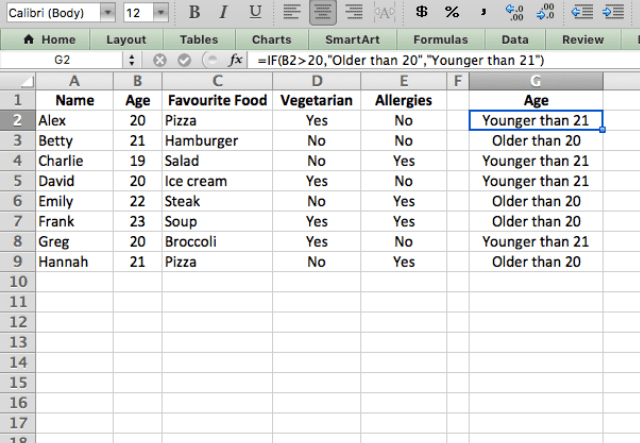Again, you can see the logic that the function is applying: If the value in the cell is greater than 20, the expression (B2>20) is TRUE, and the function outputs the value_if_true, which again is a text string. If the expression is FALSE, the function outputs the value_if_false.

I’m using the > (greater than) operator here, but you can use any of the logical operators shown below, depending on what you’re trying to accomplish.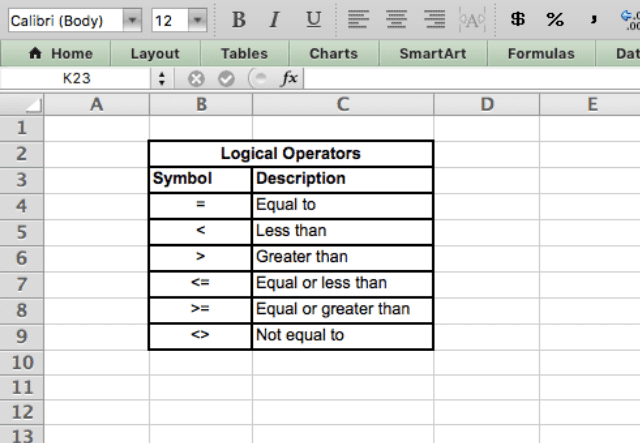#### IF function with calculations as the result

So far, the examples I’ve shown have had text strings as the outputs. But the function can also return a calculation, just like the one in the following example.

Here, I assume that the people in the spreadsheet live in a country where the legal drinking age is 21. I want to see how many years are left until they reach that age.

I do that with the formula =IF(B2<21,21-B2,”N/A”). I ask the formula if each person is younger than 21, and if the answer is yes (TRUE), then I perform a calculation to determine how many years are left until they reach the legal drinking age.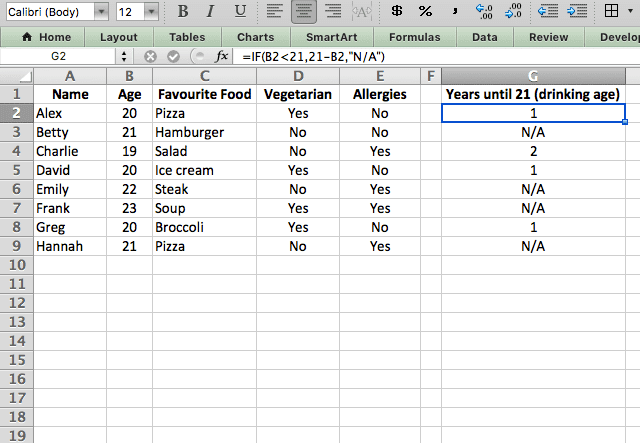### Nested IF statements

Now that you’re familiar with the basics, I’ll get into some more complex use cases of the IF function.

Often, you’ll want to use a series of IF functions in order to perform tasks that have more than two possible results. This combination of multiple IF statements (one inside the other) is called a nested IF statement.

Take a look at the following example. I want to split the data into three groups: vegetarians without allergies, vegetarians with allergies, and non-vegetarians. Because there are more than two possible outcomes, I can’t use a simple TRUE/FALSE IF function.

To overcome this, I’ve added a second IF function nested within the first. The formula I use here is =IF(D2=”Yes”,IF(E2=”No”,”Vegetarian (no allergies)”,”Vegetarian with allergies”),”No”).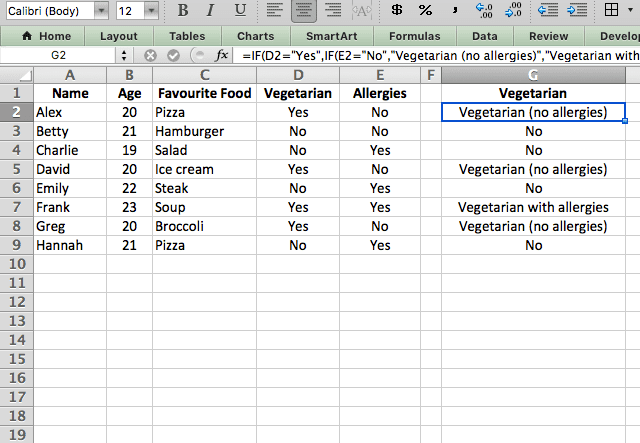The IF function starts with a simple logical expression – I ask if the person is vegetarian (cell B2 = “Yes”). But instead of just putting a value_if_true next, I’ve replaced it with another IF function.

Now look ahead to the end of the formula. You can see that after the second IF function is finished, I have my value_if_false for the first IF function. The second IF function is actually the value_if_true for the first IF function. So if the expression evaluates to Yes (in this case, if the person is vegetarian), then the function will use the second IF statement to determine what the output should be.

As you can see, this works well to give the correct answer when facing multiple possible outputs.

#### IF with AND & OR

The AND and OR functions in Excel are other logical functions that you can use together with IF. They both allow you to evaluate multiple criteria without requiring a second IF function. The AND function specifies that all the criteria have to be met, while the OR function says that at least one of multiple criteria must be met.

Take a look at the example below. Here, I use AND in combination with IF to determine which people are vegetarians with allergies. Instead of using multiple IF statements, I use the following formula: =IF(AND(D2=”Yes”,E2=”Yes”),”Yes”,”No”).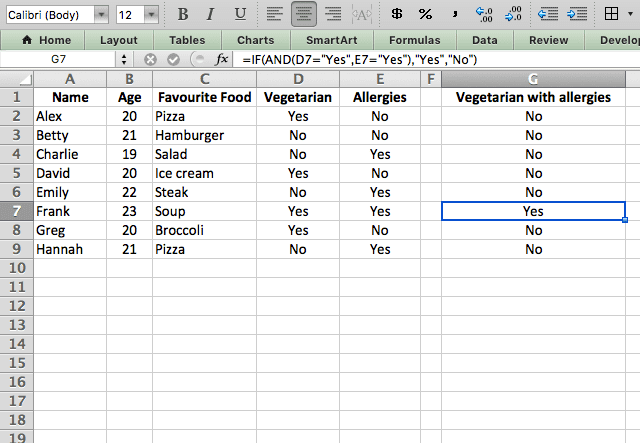As you can see, my logical expression here is AND(D2=”Yes”,E2=”Yes”). To meet the criteria TRUE, each person must have a Yes under both the vegetarian and allergies columns. If either column has a No, then the function evaluates as FALSE.

Lastly, I’ll show an OR function combined with IF. Here, I’m looking for everyone that is either a vegetarian or has allergies. I use =IF(OR(D2=”Yes”,E2=”Yes”),”Yes”,”No”) and I can see that only Betty is none of the two – everyone else has at least one “Yes”.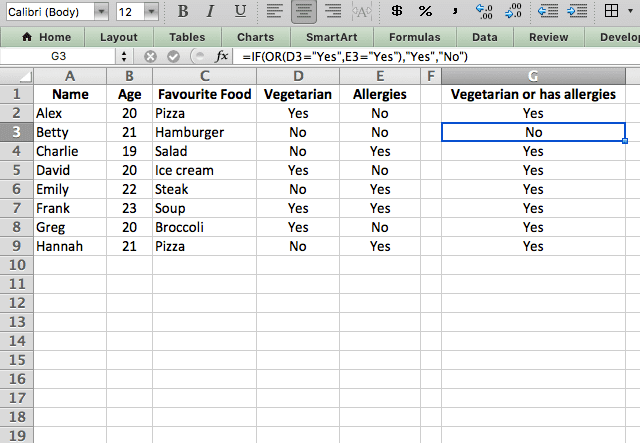### IF function in Excel

The IF function in Excel is an extremely powerful and versatile function. If you can master it, you can get your spreadsheet to do almost anything!